# Percent Composition Chapter 10 Percent Composition and Chemical

• Slides: 13Percent CompositionChapter 10 Percent Composition and Chemical Formulas • OBJECTIVES: –Describe how to calculate the percent by mass of an element in a compound.Chapter 10 - Percent Composition and Chemical Formulas • OBJECTIVES: –Interpret an empirical formula.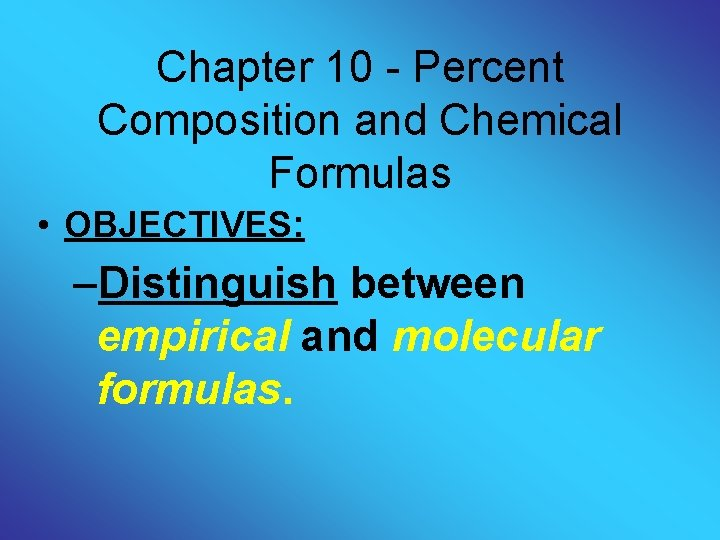Chapter 10 - Percent Composition and Chemical Formulas • OBJECTIVES: –Distinguish between empirical and molecular formulas.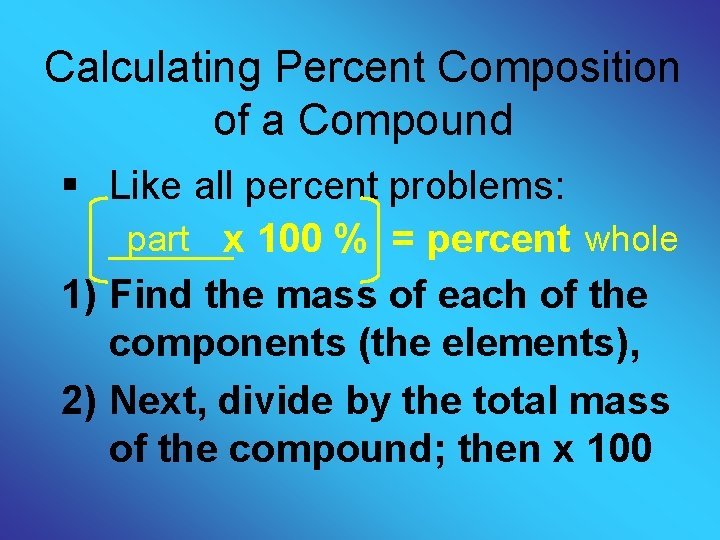Calculating Percent Composition of a Compound § Like all percent problems: part x 100 % = percent whole 1) Find the mass of each of the components (the elements), 2) Next, divide by the total mass of the compound; then x 100Example § Calculate the percent composition of a compound that is made of 29. 0 grams of Ag with 4. 30 grams of S. 29. 0 g Ag X 100 = 87. 1 % Ag 33. 3 g total 4. 30 g S X 100 = 12. 9 % S 33. 3 g total Total = 100 %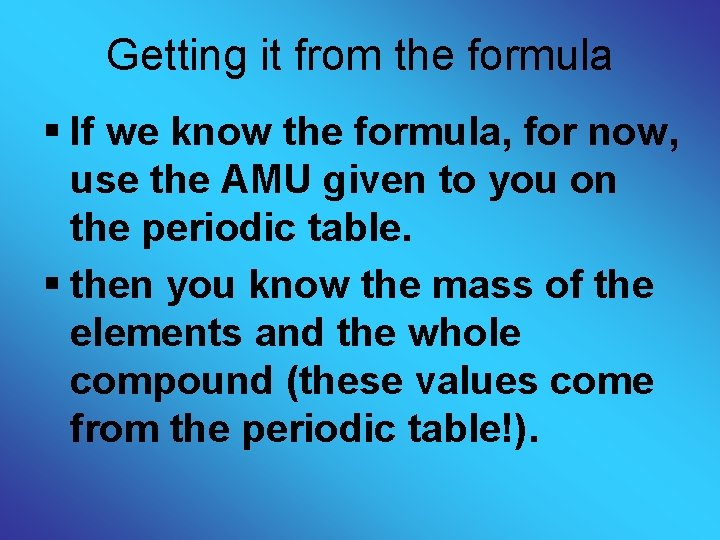Getting it from the formula § If we know the formula, for now, use the AMU given to you on the periodic table. § then you know the mass of the elements and the whole compound (these values come from the periodic table!).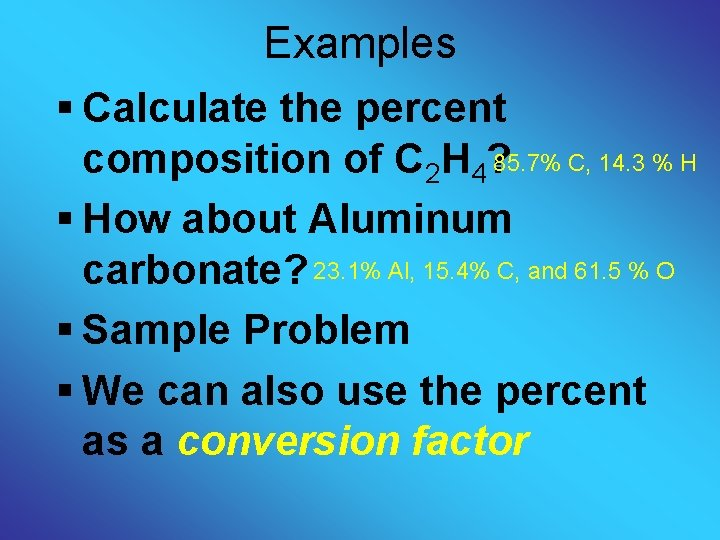Examples § Calculate the percent composition of C 2 H 4? 85. 7% C, 14. 3 % H § How about Aluminum carbonate? 23. 1% Al, 15. 4% C, and 61. 5 % O § Sample Problem § We can also use the percent as a conversion factorFormulas Empirical formula: the lowest whole number ratio of atoms in a compound. Molecular formula: the true number of atoms of each element in the formula of a compound. • Example: molecular formula for benzene is C 6 H 6 (note that everything is divisible by 6) • Therefore, the empirical formula = CH (the lowest whole number ratio)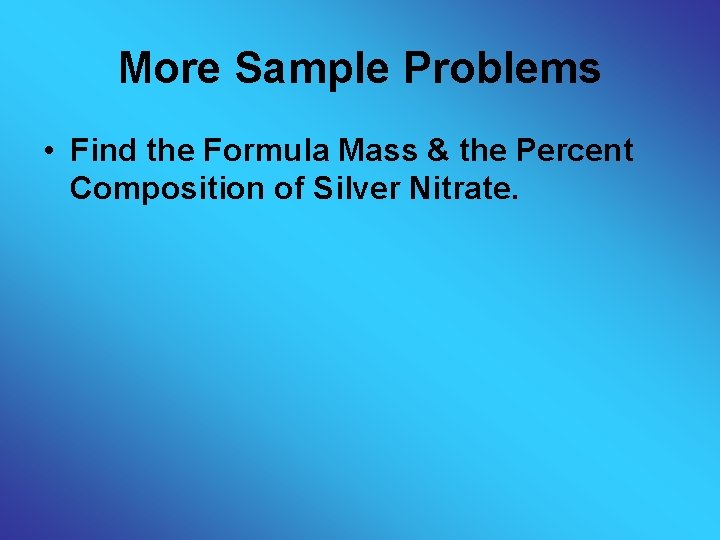More Sample Problems • Find the Formula Mass & the Percent Composition of Silver Nitrate.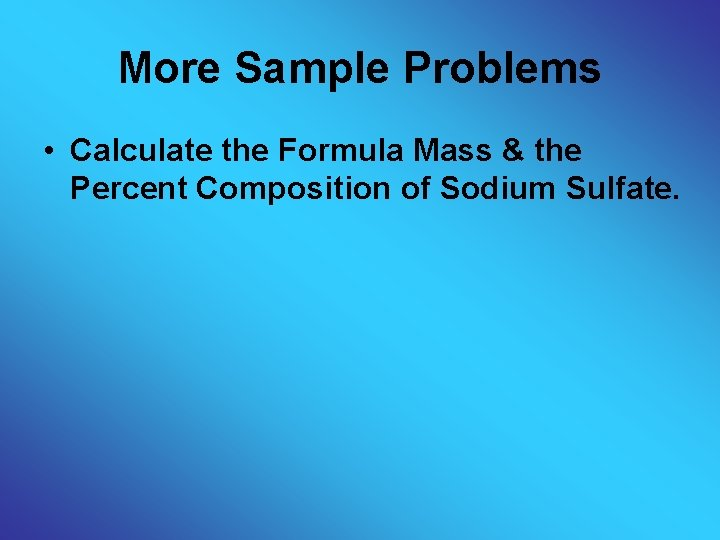More Sample Problems • Calculate the Formula Mass & the Percent Composition of Sodium Sulfate.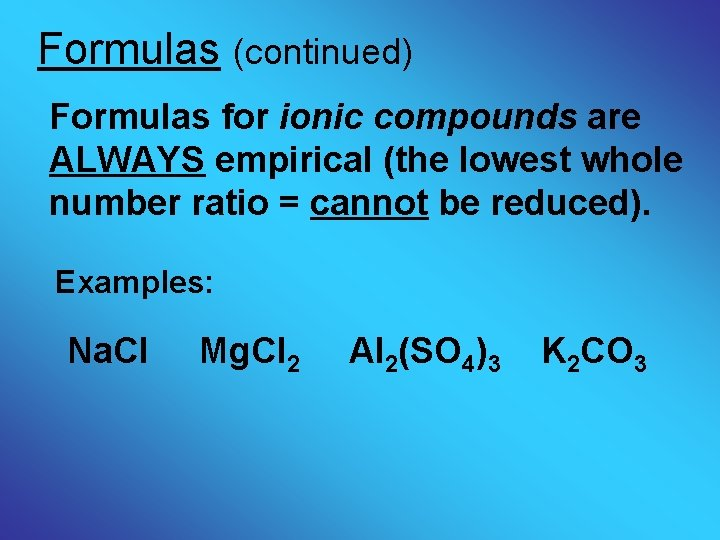Formulas (continued) Formulas for ionic compounds are ALWAYS empirical (the lowest whole number ratio = cannot be reduced). Examples: Na. Cl Mg. Cl 2 Al 2(SO 4)3 K 2 CO 3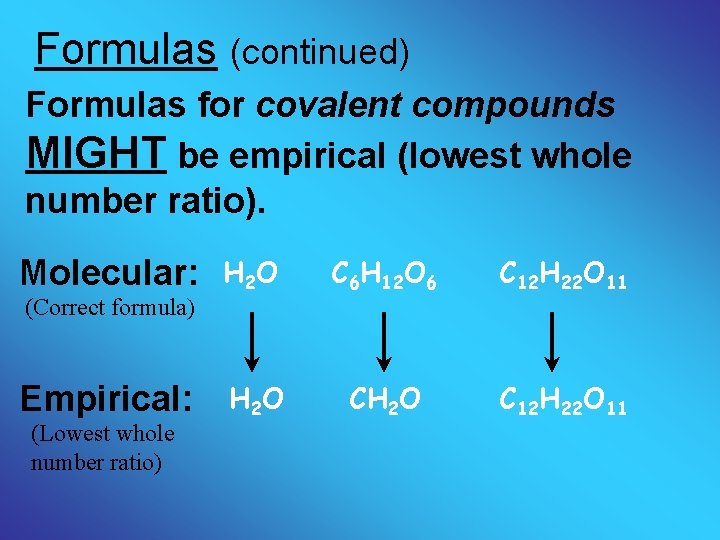Formulas (continued) Formulas for covalent compounds MIGHT be empirical (lowest whole number ratio). Molecular: (Correct formula) Empirical: (Lowest whole number ratio) H 2 O C 6 H 12 O 6 C 12 H 22 O 11 H 2 O CH 2 O C 12 H 22 O 11